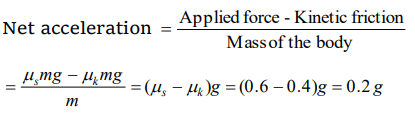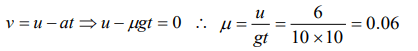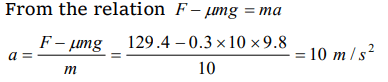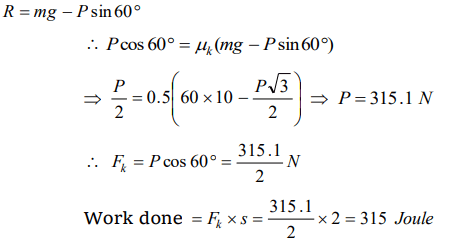## Friction Questions and Answers Part-5

1. A vehicle of mass m is moving on a rough horizontal road with momentum P. If the coefficient of friction between the tyres and the road be $\mu$, then the stopping distance is
a) $\frac{P}{2\mu mg}$
b) $\frac{P^{2}}{2\mu mg}$
c) $\frac{P}{2\mu m^{2}g}$
d) $\frac{P^{2}}{2\mu m^{2}g}$

Explanation:2. A body of weight 64 N is pushed with just enough force to start it moving across a horizontal floor and the same force continues to act afterwards. If the coefficients of static and dynamic friction are 0.6 and 0.4 respectively, the acceleration of the body will be (Acceleration due to gravity = g)
a) $\frac{g}{6.4}$
b) 0.64 g
c) $\frac{g}{32}$
d) 0.2 g

Explanation: Weight of the body = 64N
so mass of the body m = 6.4 kg , $\mu$ s = 0.6 , $\mu$ k = 0.43.When a body is moving on a surface, the force of friction is called
a) Static friction
b) Dynamic friction
c) Limiting friction
d) Rolling friction

Explanation: Dynamic friction

4. A block of mass 10 kg is placed on a rough horizontal surface having coefficient of friction $\mu$ = 0.5. If a horizontal force of 100 N is acting on it, then acceleration of the block will be
a) $0.5 m\diagup s^{2}$
b) $5 m\diagup s^{2}$
c) $10 m\diagup s^{2}$
d) $15 m\diagup s^{2}$

Explanation:5. It is easier to roll a barrel than pull it along the road. This statement is
a) False
b) True
c) Uncertain
d) Not possible

Explanation: This statement is true

6.A marble block of mass 2 kg lying on ice when given a velocity of 6 m/s is stopped by friction in 10s. Then the coefficient of friction is
a) 0.01
b) 0.02
c) 0.03
d) 0.06

Explanation:7.A horizontal force of 129.4 N is applied on a 10 kg block which rests on a horizontal surface. If the coefficient of friction is 0.3, the acceleration should be
a) 9.8 $m\diagup s^{2}$
b) 10 $m\diagup s^{2}$
c) 12.6 $m\diagup s^{2}$
d) 19.6 $m\diagup s^{2}$

Explanation:8. A 60 kg weight is dragged on a horizontal surface by a rope upto 2 metres. If coefficient of friction is $\mu=0.5$ , the angle of rope with the surface is 60° and g = $9.8 m\diagup s^{2}$ , then work done is
a) 294 joules
b) 315 joules
c) 588 joules
d) 197 joules

Explanation:9. A car having a mass of 1000 kg is moving at a speed of 30 metres/sec. Brakes are applied to bring the car to rest. If the frictional force between the tyres and the road surface is 5000 newtons, the car will come to rest in
a) 5 seconds
b) 10 seconds
c) 12 seconds
d) 6 seconds10. If $\mu_{s},\mu_{k}$  and $\mu_{r}$  are coefficients of static friction, sliding friction and rolling friction, then
a) $\mu_{s} <\mu_{k} < \mu_{r}$
b) $\mu_{k} < \mu_{r} < \mu_{s}$
c) $\mu_{r} < \mu_{k} < \mu_{s}$
d) $\mu_{r}=\mu_{k}= \mu_{s}$
Explanation: $\mu_{r} < \mu_{k} < \mu_{s}$